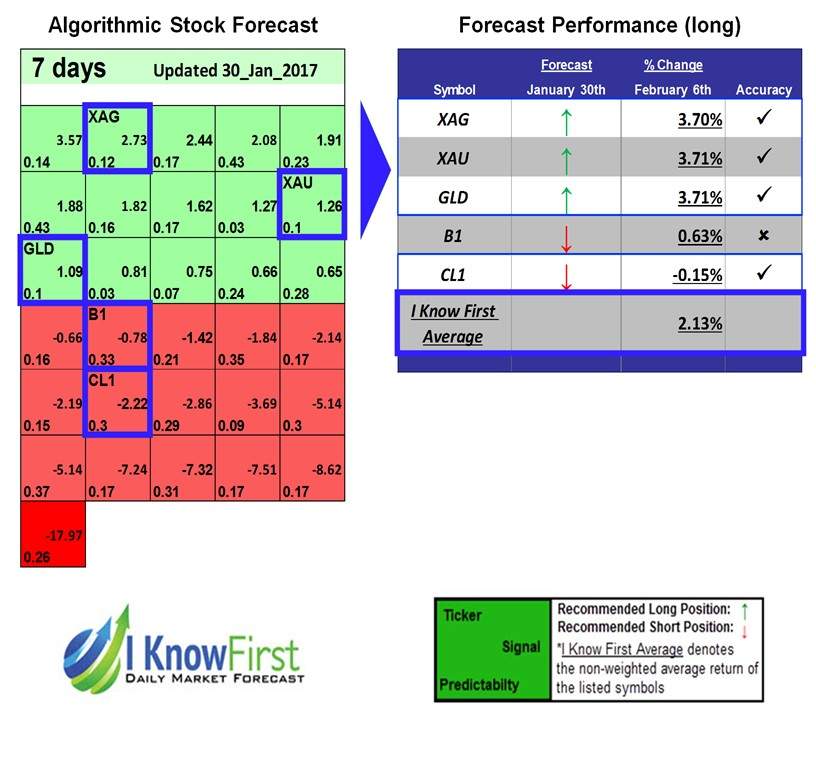Gold Forecast

This Gold forecast is part of the Commodities Package, one of I Know First’s algorithmic trading solutions. This package gives predictions for Gold and other precious metals for the various forecasted time frames and includes our algorithmic outlook for:

• Gold ETF (GLD) direction
• Physical Gold (XAU) direction
• Silver (XAG) directionPackage Name: Gold Forecast
Forecast Length: 7 Days (01/30/2017 – 02/06/2017)
I Know First Average: 2.13%How to interpret this diagram:

Algorithmic Stock Forecast: The table on the left is the stock forecast produced by I Know First’s algorithm. Each day, subscribers receive forecasts for six different time horizons. The top ten stocks in the 1-month forecast may be different than those in the 1-year forecast. In the included table, only the relevant tickers have been included. A green box represents a positive forecast while a red represents a negative forecast. The boxes are then arranged according to their respective signal and predictability values (see below for detailed definitions).

Forecast Performance: The table on the right compares the actual stock performance with I Know First’s prediction. The column titled “Forecast” shows which direction the algorithm predicted, and the column “% Change” shows the actual stock performance over the indicated time period. The “Accuracy” column shows a “v” if the algorithm correctly predicted the direction of the stock or an “x” if the forecast was incorrect. The I Know First Average is the equal-weights average percent change of the stocks listed below, and the S&P 500 may be included for reference if relevant.
Signal: This indicator represents the predicted movement direction/trend; not a percentage or specific target price. The signal strength indicates how much the current price deviates from what the system considers an equilibrium or “fair” price.
Predictability: This value is obtained by calculating the correlation between the current prediction and the actual asset movement for each discrete time period. The algorithm then averages the results of all the prediction points, while giving more weight to recent performance. As the machine keeps learning, the predictability values generally increase.